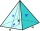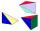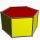# Volume + area of shape - math problems

#### Number of problems found: 135

• TerezaThe cube has an area of base 256 mm2. Calculate the edge length, volume, and area of its surface.
• The cylinder 2Find the volume and the lateral area of a cylinder of height 12 inches and a base radius of 4 inches.
• Cube 5The content area of one cube wall is 32 square centimeters. Determine the length of its edges, its surface and volume.
• The plaster castThe plaster cast has the shape of a regular quadrilateral pyramid. The cover consists of four equilateral triangles with a 5 m side. Calculate its volume and surface area.
• Hexagonal pyramidCalculate the volume and surface area of a regular hexagonal pyramid with a base edge a = 30 m and a side edge b = 50 m.
• Cuboid and eq2Calculate the volume of cuboid with square base and height 6 cm if the surface area is 48 cm2.
• A concrete pedestalA concrete pedestal has a shape of a right circular cone having a height of 2.5 feet. The diameter of the upper and lower bases are 3 feet and 5 feet, respectively. Determine the lateral surface area, total surface area, and the volume of the pedestal.
• Pentagonal pyramidThe height of a regular pentagonal pyramid is as long as the edge of the base, 20 cm. Calculate the volume and surface area of the pyramid.
• PyramidCuboid ABCDEFGH has dimensions AB 3 cm, BC 4 cm, CG 5 cm. Calculate the volume and surface area of a triangular pyramid ADEC.
• Equilateral cylinderEquilateral cylinder (height = base diameter; h = 2r) has a volume of V = 199 cm3 . Calculate the surface area of the cylinder.
• Hexaprism containerCalculate the volume and surface of a container in the shape of a regular hexagonal prism with a height of 1.4 m with a base edge of 3dm and a corresponding height of 2.6 dm.
• IcebergWhat is the surface area of 50 cm iceberg (in the shape of a cuboid) that can carry a man with luggage with a total weight of 120 kg?
• Pyramid cutWe cut the regular square pyramid with a parallel plane to the two parts (see figure). The volume of the smaller pyramid is 20% of the volume of the original one. The bottom of the base of the smaller pyramid has a content of 10 cm2. Find the area of the
• Tetrahedral pyramidCalculate the volume and surface area of a regular tetrahedral pyramid, its height is \$b cm and the length of the edges of the base is 6 cm.
• Maximum of volumeThe shell of the cone is formed by winding a circular section with a radius of 1. For what central angle of a given circular section will the volume of the resulting cone be maximum?The quadrilateral pyramid has a rectangular base of 24 cm x 3.2dm and a body height of 0.4m. Calculate its volume and surface area.
• Regular triangular pyramidCalculate the volume and surface area of the regular triangular pyramid and the height of the pyramid is 12 centimeters, the bottom edge has 4 centimeters and the height of the side wall is 12 centimeters
• Triangular pyramidIt is given perpendicular regular triangular pyramid: base side a = 5 cm, height v = 8 cm, volume V = 28.8 cm3. What is it content (surface area)?
• Tetrahedral pyramidCalculate the regular tetrahedral pyramid's volume and surface if the content area of the base is 20 cm2, and the deviation angle of the side edges from the plane of the base is 60 degrees.
• Uboid volumeCalculate the cuboid volume if the walls are 30cm², 35cm², 42cm²

Do you have an interesting mathematical word problem that you can't solve it? Submit a math problem, and we can try to solve it.

We will send a solution to your e-mail address. Solved examples are also published here. Please enter the e-mail correctly and check whether you don't have a full mailbox.

Please do not submit problems from current active competitions such as Mathematical Olympiad, correspondence seminars etc...

Tip: Our volume units converter will help you with the conversion of volume units. Volume - math word problems. Examples of area of plane shapes.